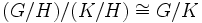Using that a subgroup is normal

This survey article is about how to use information about satisfaction of the following property: normal subgroup
Find other survey articles about normal subgroup | Find fact articles that prove satisfaction of this property

This article explores the various ways in which, given a group and a subgroup (through some kind of description) we can use the fact that the subgroup is a normal subgroup.

Ability to take the quotient group

One of the main advantages of working with normal subgroups is that we can take the quotient group of the whole group by the subgroup. Explicitly, if$H$ is a normal subgroup of a group$G$, we can consider the quotient group$G/H$. This has a number of applications:

• We might be able to use facts that we know about$H$ to deduce constraints on$G/H$.
• We might be able to deduce facts about$G$ from facts that we know about$H$ and that we deduce about$G/H$.
• This might be the template for the induction step in a proof by induction. See induction for finite groups for more.

Examples of deducing the structure of the quotient group

We have the following structural restrictions on the quotient group:

Using the isomorphism theorems

Normal subgroups set the stage for using the isomorphism theorems. The major ones are listed below.

Theorem name/number Statement
First isomorphism theorem If$\varphi:G \to H$ is a surjective homomorphism, then the kernel$N$ of$\varphi$ is a normal subgroup, and if$\alpha:G \to G/N$ is the quotient map, then there is a unique isomorphism$\psi:G/N \to H$ such that$\psi \circ \alpha = \varphi$.
Second isomorphism theorem (diamond isomorphism theorem) If$N,H \le G$ such that$N$ is contained in the normalizer of$H$, then$N$ is normal in$NH$ and$NH/N \cong H/(H \cap N)$.
Third isomorphism theorem If$H \le K \le G$ with both$H,K$ normal in$G$, then$H$ is normal in$K$ and$(G/H)/(K/H) \cong G/K$.
Fourth isomorphism theorem (lattice isomorphism theorem) If$H$ is normal in$G$, there is a bijective correspondence between subgroups of$G/H$ and subgroups of$G$ containing$H$, satisfying many nice conditions.